Search by Topic

Resources tagged with Multiplication & division similar to Extending Fraction Bars:

Filter by: Content type:
Age range:
Challenge level:

There are 137 results

Broad Topics > Calculations and Numerical Methods > Multiplication & division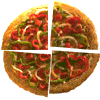Fair Feast

Age 5 to 11 Challenge Level:

Here is a picnic that Petros and Michael are going to share equally. Can you tell us what each of them will have?3388

Age 11 to 14 Challenge Level:

Using some or all of the operations of addition, subtraction, multiplication and division and using the digits 3, 3, 8 and 8 each once and only once make an expression equal to 24.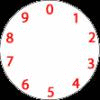Dividing a Cake

Age 7 to 11 Challenge Level:

Annie cut this numbered cake into 3 pieces with 3 cuts so that the numbers on each piece added to the same total. Where were the cuts and what fraction of the whole cake was each piece?Jumping

Age 7 to 11 Challenge Level:

After training hard, these two children have improved their results. Can you work out the length or height of their first jumps?Pies

Age 7 to 11 Challenge Level:

Grandma found her pie balanced on the scale with two weights and a quarter of a pie. So how heavy was each pie?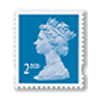Penta Post

Age 7 to 11 Challenge Level:

Here are the prices for 1st and 2nd class mail within the UK. You have an unlimited number of each of these stamps. Which stamps would you need to post a parcel weighing 825g?Napier's Bones

Age 7 to 11 Challenge Level:

The Scot, John Napier, invented these strips about 400 years ago to help calculate multiplication and division. Can you work out how to use Napier's bones to find the answer to these multiplications?Ducking and Dividing

Age 7 to 11 Challenge Level:

Your vessel, the Starship Diophantus, has become damaged in deep space. Can you use your knowledge of times tables and some lightning reflexes to survive?The Pied Piper of Hamelin

Age 7 to 11 Challenge Level:

This problem is based on the story of the Pied Piper of Hamelin. Investigate the different numbers of people and rats there could have been if you know how many legs there are altogether!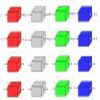Machines

Age 7 to 11 Challenge Level:

What is happening at each box in these machines?X Is 5 Squares

Age 7 to 11 Challenge Level:

Can you arrange 5 different digits (from 0 - 9) in the cross in the way described?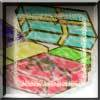Age 7 to 11 Challenge Level:

Use the information to work out how many gifts there are in each pile.Magic Potting Sheds

Age 11 to 14 Challenge Level:

Mr McGregor has a magic potting shed. Overnight, the number of plants in it doubles. He'd like to put the same number of plants in each of three gardens, planting one garden each day. Can he do it?Clock Face

Age 7 to 11 Challenge Level:

Where can you draw a line on a clock face so that the numbers on both sides have the same total?Zargon Glasses

Age 7 to 11 Challenge Level:

Zumf makes spectacles for the residents of the planet Zargon, who have either 3 eyes or 4 eyes. How many lenses will Zumf need to make all the different orders for 9 families?Sending Cards

Age 7 to 11 Challenge Level:

This challenge asks you to investigate the total number of cards that would be sent if four children send one to all three others. How many would be sent if there were five children? Six?The Deca Tree

Age 7 to 11 Challenge Level:

Find out what a Deca Tree is and then work out how many leaves there will be after the woodcutter has cut off a trunk, a branch, a twig and a leaf.A-magical Number Maze

Age 7 to 11 Challenge Level:

This magic square has operations written in it, to make it into a maze. Start wherever you like, go through every cell and go out a total of 15!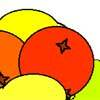Oranges and Lemons

Age 7 to 11 Challenge Level:

On the table there is a pile of oranges and lemons that weighs exactly one kilogram. Using the information, can you work out how many lemons there are?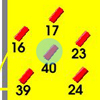Throw a 100

Age 7 to 11 Challenge Level:

Can you score 100 by throwing rings on this board? Is there more than way to do it?Divide it Out

Age 7 to 11 Challenge Level:

What is the lowest number which always leaves a remainder of 1 when divided by each of the numbers from 2 to 10?Book Codes

Age 7 to 11 Challenge Level:

Look on the back of any modern book and you will find an ISBN code. Take this code and calculate this sum in the way shown. Can you see what the answers always have in common?Number Tracks

Age 7 to 11 Challenge Level:

Ben’s class were cutting up number tracks. First they cut them into twos and added up the numbers on each piece. What patterns could they see?Fingers and Hands

Age 7 to 11 Challenge Level:

How would you count the number of fingers in these pictures?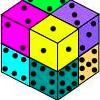Six Numbered Cubes

Age 7 to 11 Challenge Level: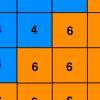Six Ten Total

Age 7 to 11 Challenge Level:

This challenge combines addition, multiplication, perseverance and even proof.Surprising Split

Age 7 to 11 Challenge Level:

Does this 'trick' for calculating multiples of 11 always work? Why or why not?What Is Ziffle?

Age 7 to 11 Challenge Level:

Can you work out what a ziffle is on the planet Zargon?Square Subtraction

Age 7 to 11 Challenge Level:

Look at what happens when you take a number, square it and subtract your answer. What kind of number do you get? Can you prove it?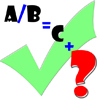Remainders

Age 7 to 11 Challenge Level:

I'm thinking of a number. When my number is divided by 5 the remainder is 4. When my number is divided by 3 the remainder is 2. Can you find my number?Escape from the Castle

Age 7 to 11 Challenge Level:

Skippy and Anna are locked in a room in a large castle. The key to that room, and all the other rooms, is a number. The numbers are locked away in a problem. Can you help them to get out?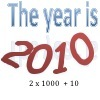It Was 2010!

Age 5 to 11 Challenge Level:

If the answer's 2010, what could the question be?Calendar Calculations

Age 7 to 11 Challenge Level:

Try adding together the dates of all the days in one week. Now multiply the first date by 7 and add 21. Can you explain what happens?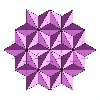Next Number

Age 7 to 11 Short Challenge Level:

Find the next number in this pattern: 3, 7, 19, 55 ...Being Resilient - Primary Number

Age 5 to 11 Challenge Level:

Number problems at primary level that may require resilience.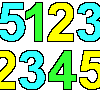Sept03 Sept03 Sept03

Age 7 to 11 Challenge Level:

This number has 903 digits. What is the sum of all 903 digits?Cows and Sheep

Age 7 to 11 Challenge Level:

Use your logical reasoning to work out how many cows and how many sheep there are in each field.Rabbits in the Pen

Age 7 to 11 Challenge Level:

Using the statements, can you work out how many of each type of rabbit there are in these pens?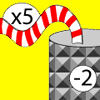Function Machines

Age 7 to 11 Challenge Level:

If the numbers 5, 7 and 4 go into this function machine, what numbers will come out?The Money Maze

Age 7 to 11 Challenge Level:

Go through the maze, collecting and losing your money as you go. Which route gives you the highest return? And the lowest?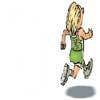Rocco's Race

Age 7 to 11 Short Challenge Level:

Rocco ran in a 200 m race for his class. Use the information to find out how many runners there were in the race and what Rocco's finishing position was.Tom's Number

Age 7 to 11 Challenge Level:

Work out Tom's number from the answers he gives his friend. He will only answer 'yes' or 'no'.Buckets of Thinking

Age 7 to 11 Challenge Level:

There are three buckets each of which holds a maximum of 5 litres. Use the clues to work out how much liquid there is in each bucket.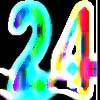Special 24

Age 7 to 11 Challenge Level:

Find another number that is one short of a square number and when you double it and add 1, the result is also a square number.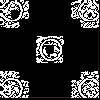Doplication

Age 7 to 11 Challenge Level:

We can arrange dots in a similar way to the 5 on a dice and they usually sit quite well into a rectangular shape. How many altogether in this 3 by 5? What happens for other sizes?Sometimes We Lose Things

Age 7 to 11 Challenge Level:

Well now, what would happen if we lost all the nines in our number system? Have a go at writing the numbers out in this way and have a look at the multiplications table.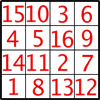Magic Constants

Age 7 to 11 Challenge Level:

In a Magic Square all the rows, columns and diagonals add to the 'Magic Constant'. How would you change the magic constant of this square?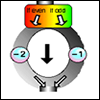The Number Crunching Machine

Age 7 to 11 Challenge Level:

Put a number at the top of the machine and collect a number at the bottom. What do you get? Which numbers get back to themselves?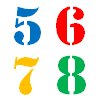How Old?

Age 7 to 11 Challenge Level:

Cherri, Saxon, Mel and Paul are friends. They are all different ages. Can you find out the age of each friend using the information?The Clockmaker's Birthday Cake

Age 7 to 11 Challenge Level:

The clockmaker's wife cut up his birthday cake to look like a clock face. Can you work out who received each piece?